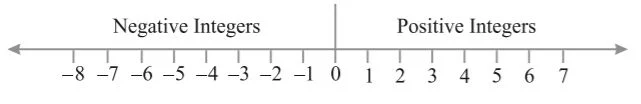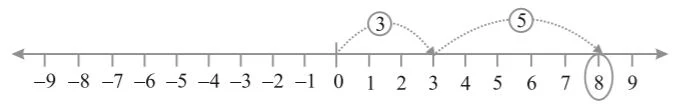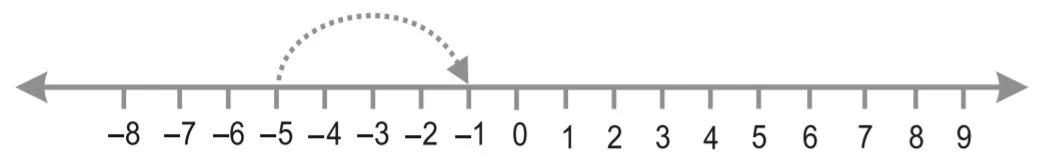# Chapter 6 Integers Class 6 Notes Maths

Chapter 6 Integers Class 6 Notes Maths will make easier for the students to comprehend the concepts due to use of easy language. Also, NCERT Solutions for Class 6 Chapter 6 Maths that will help in completing homework on time. It is quite easy to retain the answers once you are fully aware of the concept thus notes can be beneficial for you. Through these Revision notes for Class 6 a student can boost their preparation and assessment of understood concepts. It help the students in learning complex topics and chapters in an easy way.Integers

• The collection of all natural numbers, 0 and negatives of natural numbers are called integers.

Representation of integers on a number line• All the positive integers lie to the right of 0 and the negative integers to the left of 0 on the number line.

• In order to mark -5 on the number line, we move 5 points to the left of zero.

• In order to mark +5 on the number line, we move 5 points to the right of zero.

Ordering of Numbers

• On the number line, the number occurring on the right is greater than that on the left and the number on the left is smaller than that on the right.

• 2> 1, as 2 is to the right of 1.

• 0 >-2, as O is to the right of -2.

• -5>-8, as-5 is to the right of -8.

• To add two negative integers, we add the corresponding positive integers and retain the negative sign with the sum. For example: 6 + (-5) = -(6 + 5) = -11

• To add a positive integer and a negative integer, we ignore the signs and subtract integer with smaller numerical value from the integer with larger numerical value and take the sign of the larger one (a)

• Two integers whose sum is zero are called additive inverse of each other.

• To subtract an integer from a given integer, we add the additive inverse of the integer to the given integer.

Addition of Integers on a Number Line

Let us add 3 and 5 on number line.On the number line, we first move 3 steps to the right from 0 reaching 3, then we move 5 steps to the right of 3 and reach 8. Thus, we get 3 + 5 = 8.

Subtraction of Integers on a Number Line

• Subtract -4 from -5.

–5 – (–4) is same as –5 + (4), as the additive inverse of –4 is 4. We move 4 steps to the right on the number line starting from –5.